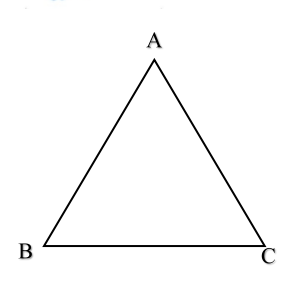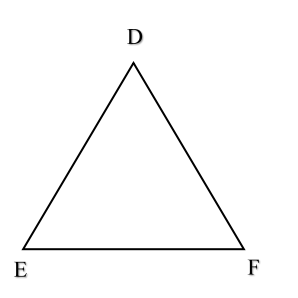Home/Class 7/Maths/

QuestionMathsClass 7

Let $$ABC$$ and $$DEF$$ be two triangles in which $$AB=DE,$$ $$BC=FD$$ and $$CA=EF.$$ The two triangles are congruent under the correspondence $$ABC\leftrightarrow$$.___

$$EDF$$
4.64.6Solution

In $$\Delta ABC$$ and $$\Delta DEF$$,.
$$AB=DE$$, (given)
$$BC=FD$$, (given)
and, $$CA=EF$$. (given)
Thus, by $$SSS$$ congruence criterion,
$$\Delta ABC\cong \Delta EDF$$.